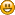###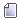Author Topic: Fafurion spawns\Schuttgart\PlunderousPlains.xml  (Read 454 times)

####Edoo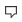##### spawns\Schuttgart\PlunderousPlains.xml
« on: October 11, 2019, 07:58:22 PM »
Code: [Select]
`<?xml version="1.0" encoding="UTF-8"?><list xmlns:xsi="http://www.w3.org/2001/XMLSchema-instance" xsi:noNamespaceSchemaLocation="../../xsd/spawns.xsd"> <spawn name="PlunderousPlains"> <group name="plunderous_plains_01"> <territories> <territory name="plunderous_plains_01" minZ="-2021" maxZ="-2199"> <node x="109480" y="-154776" /> <node x="107960" y="-155400" /> <node x="107992" y="-156824" /> <node x="105128" y="-157224" /> <node x="103912" y="-157688" /> <node x="103064" y="-159512" /> <node x="103976" y="-160472" /> <node x="104328" y="-160296" /> <node x="105576" y="-159656" /> <node x="105464" y="-157864" /> <node x="106168" y="-157816" /> <node x="107368" y="-159032" /> <node x="108136" y="-159112" /> <node x="107992" y="-158584" /> <node x="107976" y="-158120" /> <node x="108536" y="-157672" /> <node x="109416" y="-156104" /> </territory> </territories> <!-- Room 1 --> <npc id="24218" count="30" respawnTime="50sec" respawnRandom="10sec" /> <!-- Valanga Orc Scout --> <npc id="24219" count="15" respawnTime="50sec" respawnRandom="10sec" /> <!-- Valanga Orc Warrior --> <npc id="24220" count="15" respawnTime="50sec" respawnRandom="10sec" /> <!-- Valanga Orc Slaughterer --> <npc id="24221" count="30" respawnTime="50sec" respawnRandom="10sec" /> <!-- Valanga Orc Shaman --> </group> <group name="plunderous_plains_02"> <territories> <territory name="plunderous_plains_02" minZ="-1615" maxZ="-2059"> <node x="103624" y="-157304" /> <node x="101768" y="-154728" /> <node x="102904" y="-153224" /> <node x="104776" y="-153080" /> <node x="105736" y="-153816" /> <node x="106392" y="-154968" /> <node x="105912" y="-155672" /> <node x="105432" y="-155768" /> <node x="104616" y="-157080" /> </territory> </territories> <!-- Room 2 --> <npc id="24218" count="25" respawnTime="50sec" respawnRandom="10sec" /> <!-- Valanga Orc Scout --> <npc id="24219" count="10" respawnTime="50sec" respawnRandom="10sec" /> <!-- Valanga Orc Warrior --> <npc id="24220" count="10" respawnTime="50sec" respawnRandom="10sec" /> <!-- Valanga Orc Slaughterer --> <npc id="24221" count="25" respawnTime="50sec" respawnRandom="10sec" /> <!-- Valanga Orc Shaman --> </group> <group name="plunderous_plains_03"> <territories> <territory name="plunderous_plains_03" minZ="-2171" maxZ="-3071"> <node x="111288" y="-151544" /> <node x="110248" y="-151656" /> <node x="107576" y="-152728" /> <node x="105912" y="-151416" /> <node x="105496" y="-149064" /> <node x="105848" y="-147608" /> <node x="107592" y="-149096" /> <node x="108856" y="-148456" /> <node x="109448" y="-147064" /> <node x="110696" y="-147736" /> <node x="110664" y="-148920" /> <node x="110152" y="-149688" /> <node x="110792" y="-150664" /> </territory> </territories> <!-- Room 3 --> <npc id="24218" count="20" respawnTime="50sec" respawnRandom="10sec" /> <!-- Valanga Orc Scout --> <npc id="24219" count="5" respawnTime="50sec" respawnRandom="10sec" /> <!-- Valanga Orc Warrior --> <npc id="24220" count="5" respawnTime="50sec" respawnRandom="10sec" /> <!-- Valanga Orc Slaughterer --> <npc id="24221" count="20" respawnTime="50sec" respawnRandom="10sec" /> <!-- Valanga Orc Shaman --> </group> <group name="plunderous_plains_04"> <territories> <territory name="plunderous_plains_04" minZ="-3254" maxZ="-3286"> <node x="115752" y="-150600" /> <node x="116792" y="-148808" /> <node x="118632" y="-148120" /> <node x="119000" y="-148680" /> <node x="118776" y="-149576" /> <node x="118136" y="-149352" /> <node x="117464" y="-151064" /> <node x="116216" y="-151096" /> </territory> </territories> <!-- Room 4 --> <npc id="24218" count="10" respawnTime="50sec" respawnRandom="10sec" /> <!-- Valanga Orc Scout --> <npc id="24219" count="5" respawnTime="50sec" respawnRandom="10sec" /> <!-- Valanga Orc Warrior --> <npc id="24220" count="5" respawnTime="50sec" respawnRandom="10sec" /> <!-- Valanga Orc Slaughterer --> <npc id="24221" count="10" respawnTime="50sec" respawnRandom="10sec" /> <!-- Valanga Orc Shaman --> </group> <group name="plunderous_plains_05"> <territories> <territory name="plunderous_plains_05" minZ="-2734" maxZ="-3283"> <node x="120392" y="-147624" /> <node x="121448" y="-147272" /> <node x="123912" y="-145496" /> <node x="125208" y="-145624" /> <node x="124952" y="-148200" /> <node x="125544" y="-149608" /> <node x="125368" y="-150248" /> <node x="125704" y="-150760" /> <node x="126744" y="-151656" /> <node x="125448" y="-152568" /> <node x="124856" y="-151272" /> <node x="123592" y="-150872" /> <node x="123416" y="-151640" /> <node x="125544" y="-154632" /> <node x="124664" y="-155064" /> <node x="122824" y="-153368" /> <node x="120616" y="-152936" /> <node x="121207" y="-151906" /> <node x="121048" y="-148208" /> <node x="120536" y="-148168" /> </territory> </territories> <!-- Room 5 --> <npc id="24218" count="30" respawnTime="50sec" respawnRandom="10sec" /> <!-- Valanga Orc Scout --> <npc id="24219" count="15" respawnTime="50sec" respawnRandom="10sec" /> <!-- Valanga Orc Warrior --> <npc id="24220" count="15" respawnTime="50sec" respawnRandom="10sec" /> <!-- Valanga Orc Slaughterer --> <npc id="24221" count="30" respawnTime="50sec" respawnRandom="10sec" /> <!-- Valanga Orc Shaman --> </group> <group name="plunderous_plains_06"> <territories> <territory name="plunderous_plains_06" minZ="-3607" maxZ="-3660"> <node x="108808" y="-145640" /> <node x="107080" y="-146824" /> <node x="106040" y="-146056" /> <node x="105768" y="-145144" /> <node x="106456" y="-144568" /> <node x="106632" y="-143896" /> <node x="105032" y="-143608" /> <node x="104536" y="-142760" /> <node x="102504" y="-141192" /> <node x="101992" y="-141720" /> <node x="101288" y="-144392" /> <node x="99224" y="-144088" /> <node x="99256" y="-142248" /> <node x="98680" y="-140968" /> <node x="100936" y="-139624" /> <node x="101960" y="-138792" /> <node x="103400" y="-139032" /> <node x="104392" y="-140728" /> <node x="106264" y="-142584" /> <node x="107064" y="-143032" /> <node x="107368" y="-143768" /> <node x="108216" y="-144312" /> <node x="109256" y="-144536" /> <node x="109720" y="-145112" /> </territory> </territories> <!-- Room 6 --> <npc id="24218" count="30" respawnTime="50sec" respawnRandom="10sec" /> <!-- Valanga Orc Scout --> <npc id="24219" count="15" respawnTime="50sec" respawnRandom="10sec" /> <!-- Valanga Orc Warrior --> <npc id="24220" count="15" respawnTime="50sec" respawnRandom="10sec" /> <!-- Valanga Orc Slaughterer --> <npc id="24221" count="30" respawnTime="50sec" respawnRandom="10sec" /> <!-- Valanga Orc Shaman --> </group> <group name="plunderous_plains_07"> <territories> <territory name="plunderous_plains_07" minZ="-3251" maxZ="-3264"> <node x="114696" y="-149432" /> <node x="112840" y="-148264" /> <node x="110792" y="-147080" /> <node x="111208" y="-146616" /> <node x="112840" y="-147304" /> <node x="114584" y="-147800" /> <node x="114824" y="-149192" /> </territory> </territories> <!-- Room 7 --> <npc id="24218" count="10" respawnTime="50sec" respawnRandom="10sec" /> <!-- Valanga Orc Scout --> <npc id="24219" count="5" respawnTime="50sec" respawnRandom="10sec" /> <!-- Valanga Orc Warrior --> <npc id="24220" count="5" respawnTime="50sec" respawnRandom="10sec" /> <!-- Valanga Orc Slaughterer --> <npc id="24221" count="10" respawnTime="50sec" respawnRandom="10sec" /> <!-- Valanga Orc Shaman --> </group> <group name="plunderous_plains_08"> <territories> <territory name="plunderous_plains_08" minZ="-1777" maxZ="-2415"> <node x="121032" y="-154216" /> <node x="120024" y="-153768" /> <node x="119080" y="-155080" /> <node x="120440" y="-155320" /> <node x="121176" y="-155784" /> <node x="121944" y="-155656" /> <node x="122712" y="-156152" /> <node x="120536" y="-159496" /> <node x="116888" y="-158312" /> <node x="115608" y="-157800" /> <node x="115832" y="-156216" /> <node x="117448" y="-155736" /> <node x="118296" y="-155288" /> <node x="118504" y="-153576" /> <node x="119208" y="-152872" /> <node x="121192" y="-153192" /> </territory> </territories> <!-- Room 8 --> <npc id="24218" count="25" respawnTime="50sec" respawnRandom="10sec" /> <!-- Valanga Orc Scout --> <npc id="24219" count="15" respawnTime="50sec" respawnRandom="10sec" /> <!-- Valanga Orc Warrior --> <npc id="24220" count="15" respawnTime="50sec" respawnRandom="10sec" /> <!-- Valanga Orc Slaughterer --> <npc id="24221" count="25" respawnTime="50sec" respawnRandom="10sec" /> <!-- Valanga Orc Shaman --> </group> <group name="plunderous_plains_09"> <territories> <territory name="plunderous_plains_09" minZ="-957" maxZ="-1386"> <node x="120936" y="-161976" /> <node x="120792" y="-162728" /> <node x="119448" y="-163672" /> <node x="118120" y="-162808" /> <node x="117752" y="-161880" /> <node x="116792" y="-162216" /> <node x="116600" y="-161304" /> <node x="115848" y="-159848" /> <node x="116792" y="-159672" /> <node x="117128" y="-159592" /> <node x="120696" y="-160440" /> <node x="120904" y="-161176" /> </territory> </territories> <!-- Room 9 --> <npc id="24218" count="20" respawnTime="50sec" respawnRandom="10sec" /> <!-- Valanga Orc Scout --> <npc id="24219" count="10" respawnTime="50sec" respawnRandom="10sec" /> <!-- Valanga Orc Warrior --> <npc id="24220" count="10" respawnTime="50sec" respawnRandom="10sec" /> <!-- Valanga Orc Slaughterer --> <npc id="24221" count="20" respawnTime="50sec" respawnRandom="10sec" /> <!-- Valanga Orc Shaman --> </group> <group name="plunderous_plains_10"> <territories> <territory name="plunderous_plains_10" minZ="-1615" maxZ="-2020"> <node x="109800" y="-157640" /> <node x="109128" y="-158312" /> <node x="109480" y="-159752" /> <node x="110408" y="-160232" /> <node x="109944" y="-161816" /> <node x="110056" y="-162728" /> <node x="109096" y="-163784" /> <node x="107816" y="-163720" /> <node x="107481" y="-162555" /> <node x="107608" y="-161320" /> <node x="107064" y="-160200" /> <node x="105848" y="-160344" /> <node x="105224" y="-158488" /> <node x="107640" y="-158520" /> <node x="108936" y="-157432" /> </territory> </territories> <!-- Room 10 --> <npc id="24218" count="20" respawnTime="50sec" respawnRandom="10sec" /> <!-- Valanga Orc Scout --> <npc id="24219" count="10" respawnTime="50sec" respawnRandom="10sec" /> <!-- Valanga Orc Warrior --> <npc id="24220" count="10" respawnTime="50sec" respawnRandom="10sec" /> <!-- Valanga Orc Slaughterer --> <npc id="24221" count="20" respawnTime="50sec" respawnRandom="10sec" /> <!-- Valanga Orc Shaman --> </group> <group name="plunderous_plains_11"> <territories> <territory name="plunderous_plains_11" minZ="-2683" maxZ="-3154"> <node x="104856" y="-149328" /> <node x="103624" y="-149464" /> <node x="101352" y="-151320" /> <node x="100216" y="-150088" /> <node x="100264" y="-148520" /> <node x="99256" y="-147688" /> <node x="99208" y="-146744" /> <node x="98744" y="-145400" /> <node x="101656" y="-145016" /> <node x="102984" y="-144280" /> <node x="104808" y="-146136" /> <node x="105448" y="-147336" /> <node x="105656" y="-148008" /> <node x="105432" y="-149320" /> </territory> </territories> <!-- Room 11 --> <npc id="24218" count="20" respawnTime="50sec" respawnRandom="10sec" /> <!-- Valanga Orc Scout --> <npc id="24219" count="10" respawnTime="50sec" respawnRandom="10sec" /> <!-- Valanga Orc Warrior --> <npc id="24220" count="10" respawnTime="50sec" respawnRandom="10sec" /> <!-- Valanga Orc Slaughterer --> <npc id="24221" count="20" respawnTime="50sec" respawnRandom="10sec" /> <!-- Valanga Orc Shaman --> </group> </spawn></list>`
I apologize for my English, I write through Google translator.

####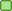Mobius

• Distinguished King
•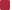•• Posts: 7212##### Re: spawns\Schuttgart\PlunderousPlains.xml
« Reply #1 on: October 11, 2019, 11:20:19 PM »
We have this on private, locations sniffed from retail, but not with zones.
How did you make zones?

####Edoo##### Re: spawns\Schuttgart\PlunderousPlains.xml
« Reply #2 on: October 12, 2019, 12:29:55 AM »
We have this on private, locations sniffed from retail, but not with zones.
How did you make zones?

1. On the official server I was in this zone, I watched their revival, how they object, after how much time.
2. Zones are manually selected. Since on the official server I noticed that the killed monster was reprimanded very far from the place of first standing. That is why I decided to fill this place according to the system of zones. I registered each point manually.
3. The number of monsters during visual observation corresponds to this place.
4. I also noticed that in some places there are also not many monsters, later I will decide on them.

P.S.
I apologize for such a translation. I write through google translator.
I apologize for my English, I write through Google translator.

####Edoo##### Re: spawns\Schuttgart\PlunderousPlains.xml
« Reply #3 on: October 12, 2019, 12:35:49 AM »
Settlement by the system of zones, takes less space in volume. And makes the player run around the location rather than stand in one place and kill monsters. I think this system is most suitable for settling open areas.
I apologize for my English, I write through Google translator.

####Mobius

••Thanks